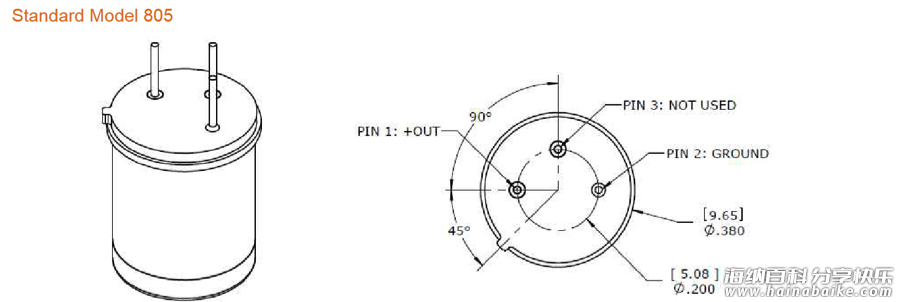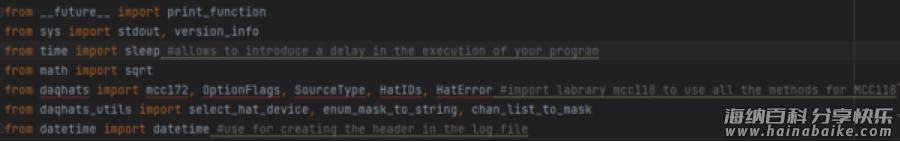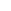# 使用 Raspberry Pi 安装的 DAQ 测量加速度计# 友情提示点击顶部放大镜 可以使用站内搜索### 如何使用安装在树莓派上的 DAQ 测量加速度计

IEPE（集成电子压电）传感器用于测量加速度、压力或力。这些类型的压电传感器包含内置的独立转换电子设备，用于将来自压电传感器的信号转换为低阻抗电压信号。

#### 所需的硬件和软件

– Raspbian OS
– GIT
– Python libraries
– MCC DAQ HAT Libraries

#### 设置必要的软件

1.如果使用图形界面，请打开终端窗口并更新您的包列表：

`sudo apt update`

2.可选：更新已安装的软件包并重新启动：

```sudo apt full-upgrade
sudo reboot
```

3.如果未安装安装 git：

`sudo apt install git`

4.使用 git 将制造商提供的软件包下载到您的用户文件夹：

```cd ~
git clone https://github.com/mccdaq/daqhats.git
```

5.安装库。安装程序将询问您是否要安装 Python 2 和 Python 3 支持。

```cd ~/daqhats
sudo ./install.sh
```

#### 设置硬件#### 程序流程

– 下载 main.py
– 下载 daqhats_utils.py (确保它与 main.py 位于同一文件夹中)

#### 导入库#### 读取和显示数据

```total_samples_read = 0
dt_string = date_time.strftime(“%d/%b/%Y/ %H:%M:%S”)#convert values store in the date_tame to string
print('

', dt_string, file=open('log.txt', 'a'))
print('
Samples Read    Scan Count       Channel 0       Channel 1', file=open('log.txt', 'a'))
timeout = 5.0
while True:
# Check for an overrun error
print('

Hardware overrun
')
break
print('

Buffer overrun
')
break
# Display the last sample for each channel,in a maximum number of 12 digits.
print('
print('
' {:12} '.format(total_samples_read), end='', file=open('log.txt', 'a'))
#
is use to write over the existing line
# Display the RMS voltage for each channel.
for i in range(num_channels):
# Display the value number of 10 digits and 5 digits after the comma
print('{:10.5f}'.format(value), 'Vrms ', end=”)
# save on a log file the value number of 10 digits and 5 digits after the comma
print('{:10.5f}'.format(value), 'Vrms ', end=”, file=open('log.txt', 'a'))
stdout.flush()
sleep(0.1)
print('
')
```

```samples_read_per_channel = int(len(read_result.data) / num_channels)
```

```f samples_read_per_channel > 0:
for i in range(num_channels):
# Display the value number of 10 digits and 5 digits after the comma
print('{:10.5f}'.format(value), 'Vrms ', end='')
# save on a log file the value number of 10 digits and 5 digits after the comma
print('{:10.5f}'.format(value), 'Vrms ', end=”, file=open('log.txt', 'a'))
```

#### 数值显示格式

```def calc_rms(data, channel, num_channels, num_samples_per_channel): “”” Calculate RMS value from a block of samples. “””
value = 0.0
index = channel
for _i in range(num_samples_per_channel):
value += (data[index]* data[index]) / num_samples_per_channel
index += num_channels
return sqrt(value)
```

```for i in range(num_channels):
```

#### 启动脚本

`python main.py`– Scan Count 是读取的样本总数，当缓冲区已满时它不会重置并继续计数。
– 通道0 和通道1 是该通道读取的值。

### 目录[+]